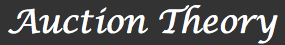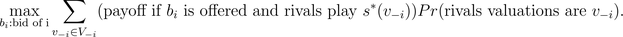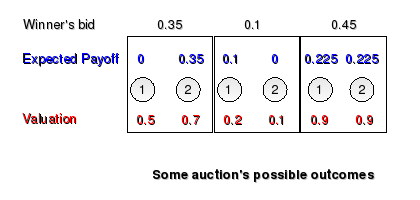Introduction1. Why study auctions? 2. The English and second-price sealed bid auctions 3. The Dutch and first-price  sealed bid auctions 4. The Revenue Equivalence  Theorem and further readingDutch and first-price sealed-bid auctions

## Dutch auction

It is important to point out that eBay uses the term "dutch auction" differently. eBay's Dutch auction is a multi-unit auction for several identical goods to be sold simultaneously to potentially multiple bidders.
This auction is the converse of the English auction. The auctioneer calls prices in a decreasing way starting from a high price. The auction ends when one bidder accepts the price. This type of auction, rather uncommon, is used, for instance, for selling cut flowers in the Netherlands, fish in Israel and tobacco in Canada.

We will see that the main difference between the equilibrium in the Dutch and English auctions is that in the Dutch auction the equilibrium strongly depends on the bidder's guess about the others decision rules, while in the English auction the bidder's strategy does not depend on what he/she believes about his/her rivals bids. To better understand this difference, it is important to define the concept of Bayesian Nash Equilibrium.

Activity 1
Organize a Dutch auction with two of your friends. Assume that their valuations satisfy the same properties as the ones listed in the example presented at the beginning of the previous lesson. You will act as the auctioneer and they will act as two bidders. Explain the rules of the game to the bidders in a clear manner. What is the outcome of the auction? How would the outcome have changed if some of the hypotheses A1-A4 are relaxed?

## Bayesian Nash Equilibrium

For simplicity we will introduce the concept in the context of auctions satisfying hypotheses A1-A4 of lesson 1. A generalization to the general case of games with incomplete information is natural. Suppose that there are N bidders in an auction, with valuations v1,...,vN. The believes of bidder i about other bidders' valuations are independent of vi and they are the same for all bidders. Let's denote by V-i the set of all possible combinations of valuations of all bidders except for bidder i. A Bayesian Nash Equilibrium is a set of strategies, (s*1,...,s*N), that specify the actions of the players given their own valuations and such that for each bidder i, the strategy s*i(vi) is on average the best response, assuming i's rivals play according to (s*1,...,s*N). In other words s*i(vi) solves the maximization problem (see figure)This definition might appear to be rather complicated because of the notation. However, a careful analysis of this concept reveals that it is a natural generalization of the concept of Nash equilibrium in games with complete information to games with incomplete information. Summarizing, in a Bayesian Nash equilibrium of an auction each bidder chooses his/her best bid given his guess about the decision rules being followed by his/her rivals (correct guess in equilibrium).

Activity 2
Explain why bidding their own valuation is a Bayesian Nash equilibrium in a second-price sealed-bid auction (see previous lesson).

It is easier to understand the outcome of a Dutch auction by studying the first-price sealed-bid auction.

## The first-price sealed-bid auction

This kind of auction has the same structure than the second-price sealed-bid auction described in the previous lesson, except that in this case the winner of the auction pays his/her own bid for the item. Unlike the English auction, bidders never see their rivals' bids and can only submit one bid. This type of auctions are commonly used for selling mineral rights to U.S government-owned land, and sometimes used for selling artwork and real estate property. Also, their analysis can be used to understand tendering of government procurement contracts.

Activity 3
1. Organize a first-price sealed-bid auction with two of your friends. Assume that their valuations satisfy the same properties as the ones listed in the example presented at the beginning of the previous lesson. You will act as the auctioneer and they will act as two bidders. Explain the rules of the game to the bidders in a clear manner. What is the outcome of the auction? How would the outcome have changed if some of the hypotheses A1-A4 are relaxed?
2. In a first-price sealed-bid auction, why is submitting bidders' own valuation is not an equilibrium anymore?

## Example

Suppose that there are only two bidders in a first-price sealed-bid auction, labeled i=1,2. The two bidders' valuations, v1, v2 are independent and uniformly distributed on [0,1]. We claim than in this case submitting vi/2 is a Bayesian Nash equilibrium for each player i (see figure below). For instance, for player 1 we have to check that v1/2 maximizes the quantity (v1-b1)Pr(b1 > v2/2), over all possible bids b1. This last expression is the expected payoff for player 1 if player 2 has the same bidding rule. From the definition of the uniform distribution this quantity is equal to (v1-b1)2b1, for b1≤ 1, and (v1-b1) for b1>1.Activity 4
Complete the reasoning initiated above. It could help to graph (v1-b1)2b1 as a function of b1 for some values of v1 in [0,1].

Hence in this case the bidder with the highest valuation wins the auction and pays a price equal to v(2)/2 (see the definition of order statistic). It turns out that the expected value of this quantity is 1/3, just like in the second-price sealed-bid auction. This is a general result that we will present in the next lesson.

We stated before that it is easier to understand the outcome of a Dutch auction by studying the first-price sealed-bid auction. This is a consequence of the following theorem.

Theorem
The Dutch auction yields the same outcome as the first-price sealed-bid auction.

It is quite easy to see why this is the case: in each auction the bidder faces the same situation, he/she has to decide how high to bid without knowing the other bidders' decisions, and if he/she wins, he/she pays a price equal to his/her own bid. Observe that this conclusion does not depend on hypotheses A1-A4.